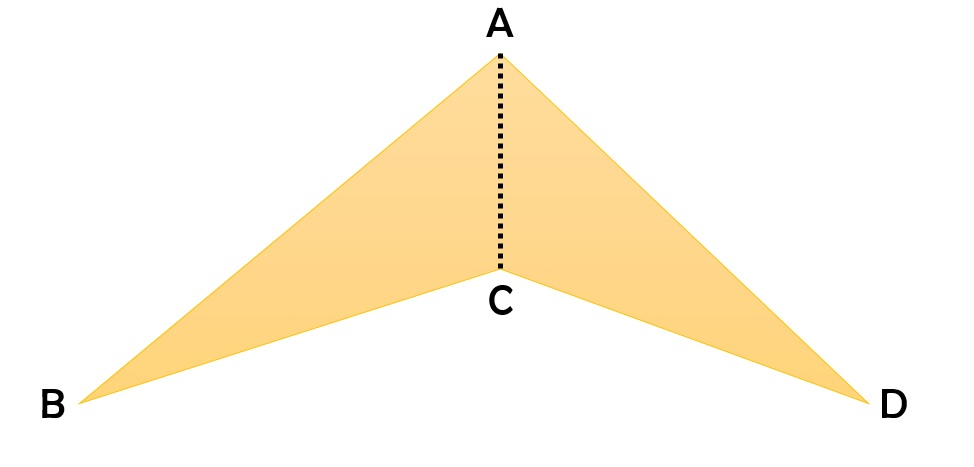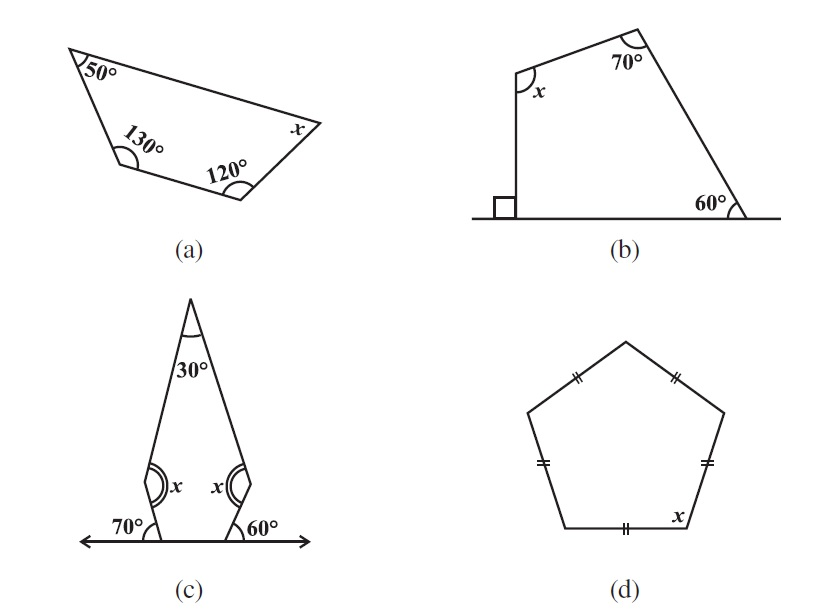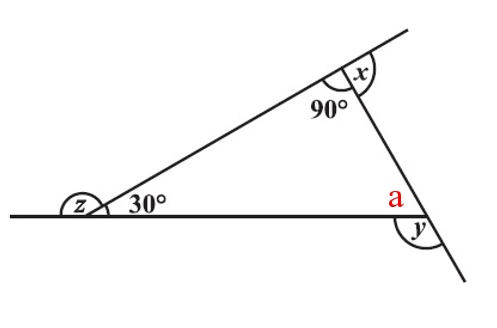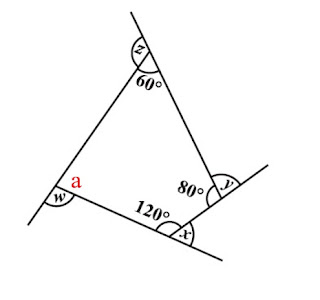# vs.eyeandcontacts.com

## Chapter 3 Understanding Quadrilaterals Exercise 3.1

Question 1: Given here are some figures.Classify each of them on the basis of the following.
a) Simple curve
b) Simple closed curve
c) Polygon
d) Convex polygon
e) Concave polygon

a) Simple curve: 1, 2, 5, 6 and 7
b) Simple closed curve: 1, 2, 5, 6 and 7
c) Polygon: 1 and 2
d) Convex polygon: 2
e) Concave polygon: 1

Question 2: How many diagonals does each of the following have?
b) A regular hexagon
c) A triangle
a)
Number of sides a convex quadrilateral has = 4
Formula for finding diagonal = n × (n - 3)
= 4 × (4 - 3)/2
= 4 × 1/2
= 4/2
= 2 diagonals

b)
Number of sides a regular hexagon has = 6
Formula for finding diagonal = n × (n - 3)
= 6 × (6 - 3)/2
= 6 × 3/2
= 18/2
= 9 diagonals

c)
Number of sides a triangle has = 3
Formula for finding diagonal = n × (n - 3)
= 3 × (3 - 3)/2
= 3 × 0/2
= 0/2
= 0 diagonals

Question 3: What is the sum of the measures of the angles of a convex quadrilateral? Will this property hold if the quadrilateral is not convex? (Make a non-convex quadrilateral and try!)
The sum of measures of the angles of a convex quadrilateral is 360°. Yes, this property will hold if the quadrilateral is not convex.Sum of all angles in a triangle = 180°
= △ABC + △ACD = ?
= 180° + 180° = 360°

Question 4: Examine the table. (Each figure is divided into triangles and the sum of the angles deduced from that.)What can you say about the angle sum of a convex polygon with number of sides?
a) 7
b) 8
c) 10
d) n

a)
Number of sides = 7
Formula for finding angle sum property = (n - 2) × 180°
= (7 - 2) × 180°
= 5 × 180°
= 900°

b)
Number of sides = 8
Formula for finding angle sum property = (n - 2) × 180°
= (8 - 2) × 180°
= 6 × 180°
= 1080°

c)
Number of sides = 10
Formula for finding angle sum property = (n - 2) × 180°
= (10 - 2) × 180°
= 8 × 180°
= 1440°

d)
Number of sides = n
Formula for finding angle sum property = (n - 2) × 180°
= (n - 2) × 180°
= (n - 2) × 180°

Question 5: What is a regular polygon?
State the name of a regular polygon of
i) 3 sides
ii) 4 sides
iii) 6 sides

Polygons which are equiangular and equilateral are called regular polygon.
i) A regular polygon of 3 sides is equilateral triangle.
ii) A regular polygon of 4 sides is square.
iii) A regular polygon of 6 sides is regular hexagon.

Question 6: Find the angle measure x in the following figures.a) Angle sum property of quadrilateral = 360°
= ∠50° + ∠130° + ∠120° + ∠x = 360°
= 300° + x = 360°
= x = 360 - 300
= x = 60°

b) Angle sum property of quadrilateral = 360°
= ∠60° + ∠70° + ∠x + ∠90° = 360°
= 220° + x = 360°
= x = 360 - 220
= x = 140°

c) Angle sum property of pentagon = 540°
= ∠30° + ∠110° + ∠120° + ∠x + ∠x = 540°
= 260° + 2x = 540°
= 2x = 540 - 260
= 2x = 280
= x = 280/2
= x = 140°

d) Angle sum property of regular pentagon = 540°
= ∠x + ∠x + ∠x + ∠x + ∠x = 540°
= 5x = 540°
= x = 540/5
= x = 108°

Question 7:a)Angle sum property of triangle = 180°
= ∠30° + ∠90° + ∠a = 180°
= 120° + a = 180°
= a = 180 - 120
= a = 60°
Therefore,
z = 180° - 30° = 150° (supplementary angles equal to 180°)
x = 180° - 90° = 90° (supplementary angles equal to 180°)
y = 180° - 60° = 120° (supplementary angles equal to 180°)

b)Angle sum property of quadrilateral = 360°
= ∠120° + ∠80° + ∠60°+ ∠a = 360°
= 260° + a = 360°
= a = 360 - 260
= a = 100°
Therefore,
w = 180° - 100° = 30° (supplementary angles equal to 180°)
x = 180° - 120° = 60° (supplementary angles equal to 180°)
y = 180° - 80° = 100° (supplementary angles equal to 180°)
z = 180° - 60° = 120° (supplementary angles equal to 180°)